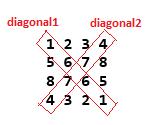### Find sum of both diagonals in matrix - program in java

Write a program to Find sum of both diagonals in matrix in java.sum of diagonal1 elements= 1+6+6+1=14,
sum of diagonal2 elements= 4+7+7+4=22
sum of diagonal1 and diagonal2 elements = 14+22= 36

Logic behind finding sum of both diagonals in matrix is:
//Logic to calculate sum of diagonal1
int sumOfDiagonal1=0;
for (int i = 0, j =0; i< rows && j < columns; i++, j++) {
sumOfDiagonal1= sumOfDiagonal1 + matrix[i][j];

}

//Logic to calculate sum of diagonal2
int sumOfDiagonal2=0;
for (int i=0,j=columns-1 ; i<rows && j>=0 ; i++, j--) {
sumOfDiagonal2= sumOfDiagonal2 + matrix[i][j];

}

Example/ Full Program/SourceCode to Find sum of both diagonals in matrix in java >
 package matrix; import java.util.Scanner; /** Copyright (c), AnkitMittal  JavaMadeSoEasy.com - Find sum of both diagonals in matrix in java */ public class SumOfBothDiagonals {    public static void main(String...args) {                     Scanner scanner = new Scanner(System.in);           System.out.print("Enter number of rows/columns in matrix : ");    //rows and columns in matrix must be same.           int rows = scanner.nextInt();           int columns=rows;           int[][] matrix = new int[rows][rows];                     System.out.println("Enter the elements in matrix :");           for (int i = 0; i < rows; i++) {                  for (int j = 0; j < columns; j++) {                        matrix[i][j] = scanner.nextInt();                  }           }           //Logic to calculate sum of diagonal1           int sumOfDiagonal1=0;           for (int i = 0, j =0; i< rows && j < columns; i++, j++) {                  sumOfDiagonal1= sumOfDiagonal1 + matrix[i][j];                            }                     //Logic to calculate sum of diagonal2           int sumOfDiagonal2=0;           for (int i=0,j=columns-1 ; i=0 ; i++, j--) {                  sumOfDiagonal2= sumOfDiagonal2 + matrix[i][j];                            }           System.out.println("\nMatrix is : ");           for (int i = 0; i < rows; i++) {              for (int j = 0; j < columns; j++) {                        System.out.print(matrix[i][j] + " ");                  }                  System.out.println();           }           System.out.println("sum of diagonal1 elements="+sumOfDiagonal1+", sum of diagonal2 elements ="+ sumOfDiagonal2 );           System.out.println("sum of diagonal1 and diagonal2 elements is: "+ (sumOfDiagonal1+ sumOfDiagonal2) );    } } /*OUTPUT Enter number of rows/columns in matrix : 4 Enter the elements in matrix : 1 2 3 4 5 6 7 8 8 7 6 5 4 3 2 1 Matrix is : 1 2 3 4 5 6 7 8 8 7 6 5 4 3 2 1 sum of diagonal1 elements=14, sum of diagonal2 elements =22 sum of diagonal1 and diagonal2 elements is: 36 */

We wrote a program to Find sum of both diagonals in matrix in java.

Having any doubt? or you you liked the tutorial! Please comment in below section.